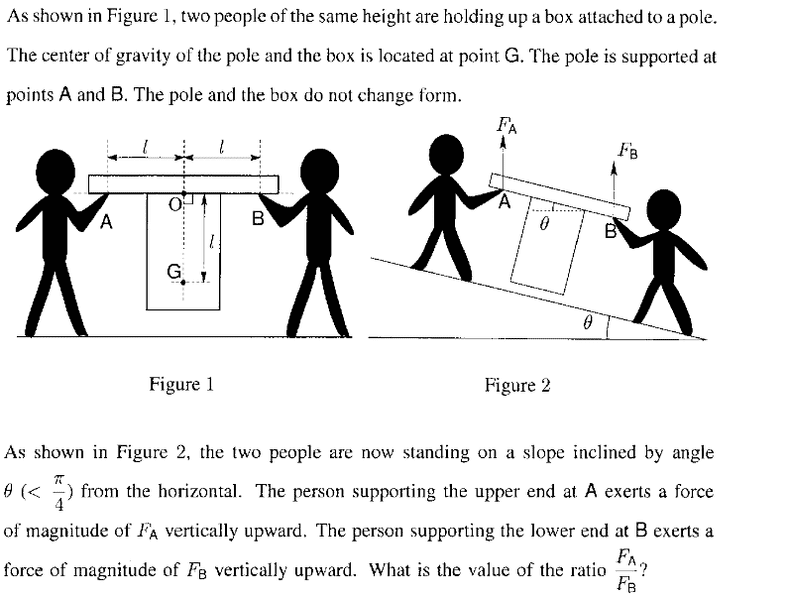# Force in Equilibrium

## Homework Statement## The Attempt at a Solution

I'm not sure about which topic it is asked. I think it's something about the centre of mass . Because the statement " The pole and the box do not change form" . The answer is
(1 + tan Θ)/(1 - tan Θ)

BvU
Homework Helper
What are (all!) the relevant equations for static equilibrium ?

Who do you think will have to carry more in the right picture ? B or A ? Why ?

The "don't change form" remark is to reassure you the center of gravity stays in the same place in the box/pole combo.

What are (all!) the relevant equations for static equilibrium ?

Who do you think will have to carry more in the right picture ? B or A ? Why ?

The "don't change form" remark is to reassure you the center of gravity stays in the same place in the box/pole combo.

A will carry more, I think it's because he's in higher position.
And is there any special formula for static equilibrium ? I just draw a triangle and use the formula F=ma.

BvU
Homework Helper
Hehe, you never had to carry something heavy down the stairs, I suppose ?

The conditions for equilibrium I hinted at in post #1 are in the first place ##\Sigma \vec F = 0## So that with your formula a = 0 ##\Rightarrow## v = constant. v = 0 remains v = 0. No translation.

But that's not enough. You also want no rotation, in other words: ##\Sigma \vec \tau = 0##.
And now the positions where the forces act come in the expressions.

Last edited:
A will carry more, I think it's because he's in higher position.
And is there any special formula for static equilibrium ? I just draw a triangle and use the formula F=ma.

F = ma comes into question when acceleration comes into question. Here, a = 0 and v = 0. All forces balance each other out. FA and FB act vertically upward. In which direction do you think 'W' acts?

DEvens